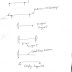Shear force and Bending moment of Beam-
Finding Difficulties While understanding shear force and bending moment?Here you'll learn this in a simplest way.

Moment of inertia:

Inertia is the property of a body by virtue of which it tends to remain in its own state i.e. at rest when still,and in motion when moving.
A force is necessary to change its state and the magnitude of the force depends upon the mass of the body.so the mass of a body is the measure of its inertia.
For a rotating body about any axis the measure of the inertia not only depends on the quantity of its mass but also on the distribution of the mass from its axis of rotation .
There are basically two types of theorem available to find out the moment of inertia.

1. Theorem of parallel axis
2. Theorem of perpendicular axis.

Moment of inertia is the name given to rotational inertia, the rotational analog of Mass for linear motion. It appears in the relationships for the dynamics of rotational motion. The moment of inertia must be specified with respect to a chosen axis of rotation. For a point mass the moment of inertia is just the mass times the square of perpendicular distance to the rotation axis, I = mr2. That point mass relationship becomes the basis for all other moments of inertia since any object can be built up from a collection of point masses.

First of all a ideal beams is required to understand sf and bm.
Beams are mainly classified in 5 type

1. cantilever
2. simply supported
3. overhanging
4. propped cantilever
5. fixed.

After talking about beam we need to know about types of loading that can occurs on a beam.

1. point load or co
2. uniformly distributed load
what is shear force and bending moment?

Shear force- The shear force at any section of a beam is the algebraic sum of the moments of all the forces taken either to the left or to the right side of the section.

Bending moment-the bending moment at any section of a beam is the algebraic sum of the moments of all the forces taken either to the left or to the right side of the section.

Shear force and bending moments are generally develop on a beam when a beam subjected to lateral
Forces if left hand sight load is in  upward direction  and right hand sight force is in downward direction.
A negative shear force is a force if a left hand sight force is in downward direction and right hand sight force is in upward direction.

Sign conventions

a).Due to shear force one portion of the beam slides upward or downward with respect to other portion.

Some important points to draw shear force and bending moment diagrams:

1. The + values of shear force and bending moment are plotted above the base line and - values below the base line.
2.For beams subjected to uniformly varying load the shear force and bending moment diagrams will be parabolic and cubic curve respectively.

I Hope you liked the basic about shear force and bending moment.Do spread the knowledge among your friends.

SHEAR FORCE AND BENDING MOMENT OF BEAMReviewed by Sk rafiqul islam on July 11, 2018 Rating: 5